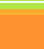Percentages of ElementsThe relative formula mass (Mr) of a compound is the sum of the relative atomic masses in the numbers shown in the formula.
The percentage of an element in a compound can be calculated from the relative mass of the element in the compound and the relative formula mass of the compound. (AQA GCSE Chemistry Unit 2)
 Composition of   () Calculate: 1. The relative formula mass ofg   2. The % composition by element (to 1 dp):  =%;   =%;   =%   =%